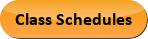Dec 03, 2023
2022-2023 College Catalog
 Select a Catalog 2023-2024 College Catalog 2022-2023 College Catalog [ARCHIVED CATALOG] 2021-2022 College Catalog [ARCHIVED CATALOG] 2020-2021 College Catalog [ARCHIVED CATALOG] 2019-2020 College Catalog [ARCHIVED CATALOG] *** DRAFT 2024-2025 College Catalog DRAFT *** [ARCHIVED CATALOG]
 HELP 2022-2023 College Catalog [ARCHIVED CATALOG] Print-Friendly Page (opens a new window) Add to Portfolio (opens a new window)

# MAT 212 - Topics in Calculus [SUN# MAT 2212]

3 Credits, 3 Contact Hours
3 lecture periods 0 lab periods

Introductory topics in differential and integral calculus to include limits, continuity, differentiation, and integration of functions with particular emphasis on business applications. Microsoft Excel and/or graphing calculators will be used as tools for further understanding of these concepts.

Prerequisite(s): Within the last three years: MAT 151  or MAT 187  or MAT 188  with a grade of C or better, or satisfactory score on the mathematics assessment exam.
Information: A graphing calculator (technology) is required. See your instructor for details.
Gen-Ed: Meets AGEC - MATH; Meets CTE - M&S.Course Learning Outcomes
1. Evaluate limits of functions.
2. Differentiate functions and apply derivatives.
3. Determine antiderivatives of functions and apply the Fundamental Theorem of Calculus.

Performance Objectives:
1. Evaluate limits of algebraic functions.
2. Use the definition to determine continuity of a function.
3. Use the definition to determine the derivative of algebraic functions.
4. Use techniques of differentiation on powers, sums, products, quotients, exponential, logarithmic, composite, and implicit functions. Calculate higher order derivatives.
5. Use the first and second derivatives to determine intervals where a function is increasing, decreasing, concave up, concave down; find points of inflection, relative and absolute extrema; and graph the function.
6. Use derivatives to solve a variety of application problems including optimization and rates of change with an emphasis in business. Explain the meaning of the derivative in the applications using appropriate units.
7. Find antiderivatives of polynomials, exponential functions and some rational functions.
8. Use finite sums to estimate the definite integral of functions defined numerically, graphically, or analytically.  Estimation techniques should include left and right hand sums.
9. Evaluate indefinite integrals.  Use the integration technique of substitution.  Use the fundamental Theorem of Calculus to evaluate definite integrals.
10. Use integration to solve applications problems including area between two curves and consumer and producer surplus.  Interpret the meaning of the integral in the applications using appropriate units.

Outline:
1. Limits
1. Definition and notation
2. Evaluation of limits
1. Continuity
1. Definition
2. Continuity at a point
3. Continuity on an interval
1. Differentiation
1. Definition of derivative
2. Rules for derivatives

1. Power rule

2. Product rule

3. Quotient rule

4. Exponential/Logarithmic rules

I. Chain Rule

1. Implicit Differentiation
2. Higher order derivatives
1. Applications of the derivative
1. Intervals of increase or decrease
2. Relative and absolute extrema
3. Concavity
4. Points of Inflection
5. Graphs of Functions
2. Mathematical modeling with the derivative
1. Optimization
2. Marginal cost, marginal revenue, marginal profit
3. Interpretation of mathematical models
3. Antiderivatives
1. Rules for antiderivatives
2. Antiderivatives of polynomial and rational functions
3. Antiderivatives of exponential functions
1. Approximation of definite integrals
1. Area under a graph
2. Left hand sum
3. Right hand sum
2. Integration
1. Indefinite integral
2. Fundamental Theorem of Calculus
3. Definite integral
1. Integration by substitution
1. Applications of integration
1. Area between two curves
2. Consumer and producer surplus
3. Interpretation of mathematical models

Add to Portfolio (opens a new window)